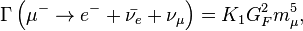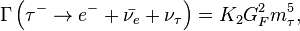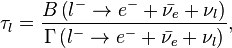# Lepton

lepton is an elementary, spin-12 particle that does not undergo strong interactions, but is subject to the Pauli exclusion principle. The best known of all leptons is the electron, which governs nearly all of chemistry as it is found in atoms and is directly tied to allchemical properties. Two main classes of leptons exist: charged leptons (also known as the electron-like leptons), and neutral leptons (better known as neutrinos). Charged leptons can combine with other particles to form various composite particles such as atoms andpositronium, while neutrinos rarely interact with anything, and are consequently rarely observed.

There are six types of leptons, known as flavours, forming three generations. The first generation is the electronic leptons, comprising the electron (e−) and electron neutrino (ν
e); the second is the muonic leptons, comprising the muon (μ−) and muon neutrino(ν
μ); and the third is the tauonic leptons, comprising the tau (τ−) and the tau neutrino (ν
τ). Electrons have the least mass of all the charged leptons. The heavier muons and taus will rapidly change into electrons through a process of particle decay: the transformation from a higher mass state to a lower mass state. Thus electrons are stable and the most common charged lepton in the universe, whereas muons and taus can only be produced in high energy collisions (such as those involving cosmic rays and those carried out inparticle accelerators).

Leptons have various intrinsic properties, including electric charge, spin, and mass. Unlike quarks however, leptons are not subject to the strong interaction, but they are subject to the other three fundamental interactions: gravitation, electromagnetism (excluding neutrinos, which are electrically neutral), and the weak interaction. For every lepton flavor there is a corresponding type of antiparticle, known as antilepton, that differs from the lepton only in that some of its properties have equal magnitude but opposite sign. However, according to certain theories, neutrinos may be their own antiparticle, but it is not currently known whether this is the case or not.

The first charged lepton, the electron, was theorized in the mid-19th century by several scientists and was discovered in 1897 byJ. J. Thomson. The next lepton to be observed was the muon, discovered by Carl D. Anderson in 1936, but it was erroneously classified as a meson at the time. After investigation, it was realized that the muon did not have the expected properties of a meson, but rather behaved like an electron, only with higher mass. It took until 1947 for the concept of “leptons” as a family of particle to be proposed. The first neutrino, the electron neutrino, was proposed by Wolfgang Pauli in 1930 to explain certain characteristics of beta decay. It was first observed in the Cowan–Reines neutrino experiment conducted by Clyde Cowan and Frederick Reines in 1956. The muon neutrino was discovered in 1962 by Leon M. Lederman, Melvin Schwartz and Jack Steinberger, and the tau discovered between 1974 and 1977 by Martin Lewis Perl and his colleagues from the Stanford Linear Accelerator Center and Lawrence Berkeley National Laboratory. The tau neutrino remained elusive until July 2000, when the DONUT collaboration from Fermilab announced its discovery.

Leptons are an important part of the Standard Model. Electrons are one of the components of atoms, alongside protons and neutrons. Exotic atoms with muons and taus instead of electrons can also be synthesized, as well as lepton–antilepton particles such as positronium.

## Contents

• 1 Etymology
• 2 History
• 3 Properties
• 3.1 Spin and chirality
• 3.2 Electromagnetic interaction
• 3.3 Weak Interaction
• 3.4 Mass
• 3.5 Leptonic numbers
• 4 Universality
• 5 Table of leptons

## Etymology

The name lepton comes from the Greek “λεπτόν” (lepton), neuter of “λεπτός” (leptos), “fine, small, thin” and the earliest attested form of the word is the Mycenaean Greek re-po-to, written in Linear B syllabic script. Lepton was first used by physicist Léon Rosenfeld in 1948:

Following a suggestion of Prof. C. Møller, I adopt — as a pendant to “nucleon” — the denomination “lepton” (from λεπτός, small, thin, delicate) to denote a particle of small mass.

The etymology incorrectly implies that all the leptons are of small mass. When Rosenfeld named them, the only known leptons were electrons and muons, which are in fact of small mass — the mass of an electron (0.511 MeV/c2) and the mass of a muon (with a value of 105.7 MeV/c2) are fractions of the mass of the “heavy” proton (938.3 MeV/c2). However, the mass of the tau (discovered in the mid 1970s) (1777 MeV/c2) is nearly twice that of the proton, and about 3,500 times that of the electron.

## HistoryA muon transmutes into a muon neutrino by emitting a W− boson. TheW− boson subsequently decays into anelectron and an electron antineutrino.

Lepton nomenclature
Particle name Antiparticle name
Electron Antielectron
Positron
Electron neutrino Electron antineutrino
Muon
Mu lepton
Mu
Antimuon
Antimu lepton
Antimu
Muon neutrino
Muonic neutrino
Mu neutrino
Muon antineutrino
Muonic antineutrino
Mu antineutrino
Tau
Tau lepton
Tauon
Antitau
Antitau lepton
Antitauon
Tau neutrino
Tauonic neutrino
Tau antineutrino
Tauonic antineutrino

The first lepton identified was the electron, discovered by J.J. Thomson and his team of British physicists in 1897. Then in 1930 Wolfgang Pauli postulated the electron neutrino to preserveconservation of energy, conservation of momentum, and conservation of angular momentum in beta decay. Pauli theorized that an undetected particle was carrying away the difference between theenergy, momentum, and angular momentum of the initial and observed final particles. The electron neutrino was simply called the neutrino, as it was not yet known that neutrinos came in different flavours (or different “generations”).

Nearly 40 years after the discovery of the electron, the muon was discovered by Carl D. Anderson in 1936. Due to its mass, it was initially categorized as a meson rather than a lepton. It later became clear that the muon was much more similar to the electron than to mesons, as muons do not undergo the strong interaction, and thus the muon was reclassified: electrons, muons, and the (electron) neutrino were grouped into a new group of particles – the leptons. In 1962 Leon M. Lederman, Melvin Schwartz and Jack Steinberger showed that more than one type of neutrino exists by first detecting interactions of themuon neutrino, which earned them the 1988 Nobel Prize, although by then the different flavours of neutrino had already been theorized.

The tau was first detected in a series of experiments between 1974 and 1977 by Martin Lewis Perl with his colleagues at the SLAC LBL group. Like the electron and the muon, it too was expected to have an associated neutrino. The first evidence for tau neutrinos came from the observation of “missing” energy and momentum in tau decay, analogous to the “missing” energy and momentum in beta decay leading to the discovery of the electron neutrino. The first detection of tau neutrino interactions was announced in 2000 by the DONUTcollaboration at Fermilab, making it the latest particle of the Standard Model to have been directly observed, apart from the Higgs boson, which probably has been discovered in 2012.

Although all present data is consistent with three generations of leptons, some particle physicists are searching for a fourth generation. The current lower limit on the mass of such a fourth charged lepton is 100.8 GeV/c2, while its associated neutrino would have a mass of at least 45.0 GeV/c2.

## Properties

### Spin and chiralityLeft-handed and right-handed helicities

Leptons are spin-12 particles. The spin-statistics theorem thus implies that they are fermions and thus that they are subject to the Pauli exclusion principle; no two leptons of the same species can be in exactly the same state at the same time. Furthermore, it means that a lepton can have only two possible spin states, namely up or down.

A closely related property is chirality, which in turn is closely related to a more easily visualized property called helicity. The helicity of a particle is the direction of its spin relative to its momentum; particles with spin in the same direction as their momentum are called right-handed and otherwise they are called left-handed. When a particle is mass-less, the direction of its momentum relative to its spin is frame independent, while for massive particles it is possible to ‘overtake’ the particle by a Lorentz transformation flipping the helicity. Chirality is a technical property (defined through the transformation behaviour under the Poincaré group) that agrees with helicity for (approximately) massless particles and is still well defined for massive particles.

In many quantum field theories—such as quantum electrodynamics and quantum chromodynamics—left and right-handed fermions are identical. However in the Standard Model left-handed and right-handed fermions are treated asymmetrically. Only left-handed fermions participate in the weak interaction, while there are no right-handed neutrinos. This is an example of parity violation. In the literature left-handed fields are often denoted by a capital L subscript (e.g. e−L) and right-handed fields are denoted by a capital R subscript.

### Electromagnetic interactionLepton-photon interaction

One of the most prominent properties of leptons is their electric charge, Q. The electric charge determines the strength of their electromagnetic interactions. It determines the strength of the electric field generated by the particle (see Coulomb’s law) and how strongly the particle reacts to an external electric or magnetic field (see Lorentz force). Each generation contains one lepton with Q = −1 (conventionally the charge of a particle is expressed in units of the elementary charge) and one lepton with zero electric charge. The lepton with electric charge is commonly simply referred to as a ‘charged positive lepton’ while the neutral lepton is called a neutrino. For example the first generation consists of the electron e− with a negative electric charge and the electrically neutral electron neutrino ν
e.

In the language of quantum field theory the electromagnetic interaction of the charged leptons is expressed by the fact that the particles interact with the quantum of the electromagnetic field, the photon. The Feynman diagram of the electron-photon interaction is shown on the right.

Because leptons possess an intrinsic rotation in the form of their spin, charged leptons generate a magnetic field. The size of theirmagnetic dipole moment μ is given by,where m is the mass of the lepton and g is the so-called g-factor for the lepton. First order approximation quantum mechanics predicts that the g-factor is 2 for all leptons. However, higher order quantum effects caused by loops in Feynman diagrams introduce corrections to this value. These corrections, referred to as the anomalous magnetic dipole moment, are very sensitive to the details of a quantum field theory model and thus provide the opportunity for precision tests of the standard model. The theoretical and measured values for the electron anomalous magnetic dipole moment are within agreement within eight significant figures.

### Weak InteractionThe weak interactions of the first generation leptons.

In the Standard Model the left-handed charged lepton and the left-handed neutrino are arranged in doublet (ν
eL, e−L) that transforms in the spinor representation (T = 12) of the weak isospinSU(2) gauge symmetry. This means that these particles are eigenstates of the isospin projection T3 with eigenvalues 12 and −12 respectively. In the meantime, the right-handed charged lepton transforms as a weak isospin scalar (T = 0) and thus does not participate in the weak interaction, while there is no right-handed neutrino at all.

The Higgs mechanism recombines the gauge fields of the weak isospin SU(2) and the weak hypercharge U(1) symmetries to three massive vector bosons (W+, W−, Z0) mediating the weak interaction, and one massless vector boson, the photon, responsible for the electromagnetic interaction. The electric charge Q can be calculated from the isospin projection T3 and weak hypercharge YW through the Gell-Mann–Nishijima formula,

Q = T3 + YW/2

To recover the observed electric charges for all particles the left-handed weak isospin doublet (ν
eL, e−L) must thus have YW = −1, while the right-handed isospin scalar e−
R must haveYW = −2. The interaction of the leptons with the massive weak interaction vector bosons is shown in the figure on the left.

### Mass

In the Standard Model each lepton starts out with no intrinsic mass. The charged leptons (i.e. the electron, muon, and tau) obtain an effective mass through interaction with theHiggs field, but the neutrinos remain massless. For technical reasons the masslessness of the neutrinos implies that there is no mixing of the different generations of charged leptons as there is for quarks. This is in close agreement with current experimental observations.

It is however known from experiment – most prominently from observed neutrino oscillations – that neutrinos do in fact have some very small mass, probably less than2 eV/c2. This implies that there are physics beyond the Standard Model. The currently most favoured extension is the so-called seesaw mechanism, which would explain both why the left-handed neutrinos are so light compared to the corresponding charged leptons, and why we have not yet seen any right-handed neutrinos.

### Leptonic numbers

The members of each generation’s weak isospin doublet are assigned leptonic numbers that are conserved under the Standard Model. Electrons and electron neutrinos have anelectronic number of Le = 1, while muons and muon neutrinos have a muonic number of Lμ = 1, while tau particles and tau neutrinos have a tauonic number of Lτ = 1. The antileptons have their respective generation’s leptonic numbers of −1.

Conservation of the leptonic numbers means that the number of leptons of the same type remains the same, when particles interact. This implies that leptons and antileptons must be created in pairs of a single generation. For example, the following processes are allowed under conservation of leptonic numbers:Each generation forms a weak isospindoublet.

e− + e+ → γ + γ,
τ− + τ+ → Z0 + Z0,

but not these:

γ → e− + μ+,
W− → e− + ν
τ,
Z0 → μ− + τ+.

However, neutrino oscillations are known to violate the conservation of the individual leptonic numbers. Such a violation is considered to be smoking gun evidence for physics beyond the Standard Model. A much stronger conservation law is the conservation of the total number of leptons (L), conserved even in the case of neutrino oscillations, but even it is still violated by a tiny amount by the chiral anomaly.

## Universality

The coupling of the leptons to gauge bosons are flavour-independent (i.e., the interactions between leptons and gauge bosons are the same for all leptons). This property is called lepton universality and has been tested in measurements of the tau and muon lifetimes and of Z boson partial decay widths, particularly at the Stanford Linear Collider (SLC) and Large Electron-Positron Collider (LEP) experiments.

The decay rate (Γ) of muons through the process μ− → e− + ν
e + ν
μ is approximately given by an expression of the form (see muon decay for more details)where K1 is some constant, and GF is the Fermi coupling constant. The decay rate of tau particles through the process τ− → e− + ν
e + ν
τ is given by an expression of the same formwhere K2 is some constant. Electron–muon universality implies that K1 = K2, and thusUniversality also accounts for the ratio of muon and tau lifetimes. The lifetime of a lepton (τl) is related to the decay rate bywhere B(x → y) and Γ(x → y) denotes the branching ratios and the resonance width of the process x → y.

The ratio of tau and muon lifetime is thus given byUsing the values of the 2008 Review of Particle Physics for the branching ratios of muons and tau yields a lifetime ratio of ~1.29×10−7, comparable to the measured lifetime ratio of ~1.32×10−7. The difference is due to K1 and K2 not actually being constants; they depend on the mass of leptons.

## Table of leptons

Properties of leptons
Particle/Antiparticle Name Symbol Q (e) S Le Lμ Lτ Mass (MeV/c2) Lifetime (s) Common decay
Electron / Positron e−/e+ −1/+1 12 +1/−1 0 0 0.510998910(13) Stable Stable
Muon / Antimuon μ−/μ+ −1/+1 12 0 +1/−1 0 105.6583668(38) 2.197019(21)×10−6 e− + ν
e + ν
μ
Tau / Antitau τ−/τ+ −1/+1 12 0 0 +1/−1 1776.84(17) 2.906(10)×10−13 See τ− decay modes
Electron neutrino / Electron antineutrino ν
e/ν
e
0 12 +1/−1 0 0 < 0.0000022 Unknown
Muon neutrino / Muon antineutrino ν
μ/ν
μ
0 12 0 +1/−1 0 < 0.17 Unknown
Tau neutrino / Tau antineutrino ν
τ/ν
τ
0 12 0 0 +1/−1 < 15.5 Unknown
• Koide formula
• List of particles
• Quark
• Preons – Hypothetical particles which were once postulated to be subcomponents of quarks and leptons

Source: Wikipedia